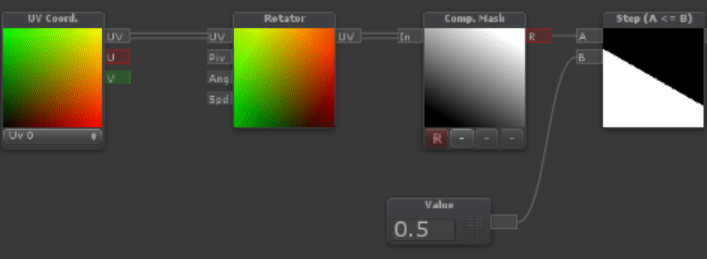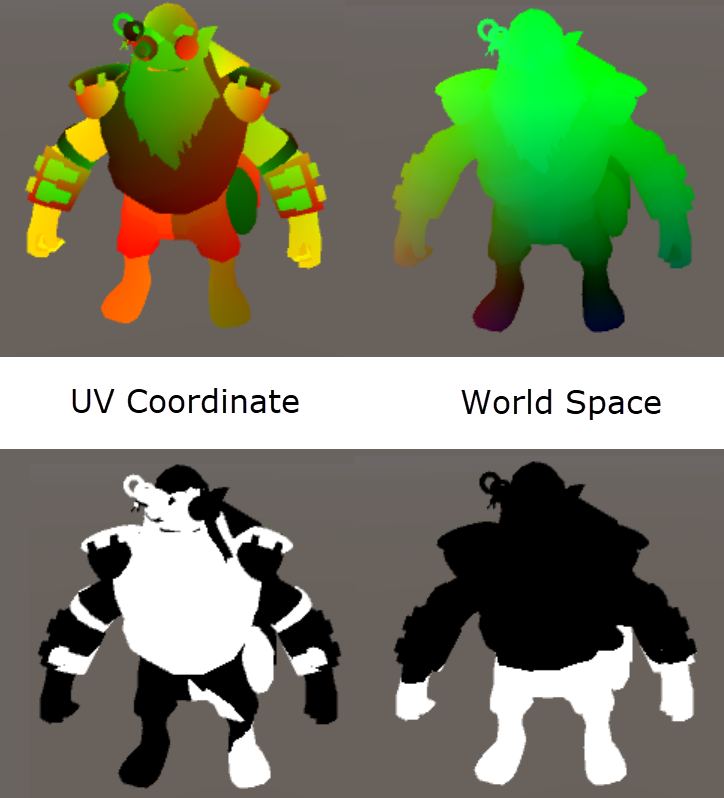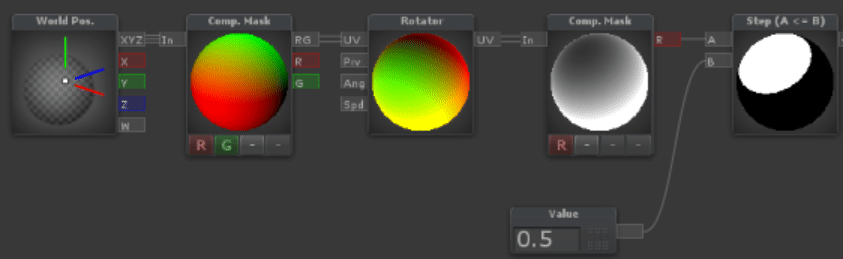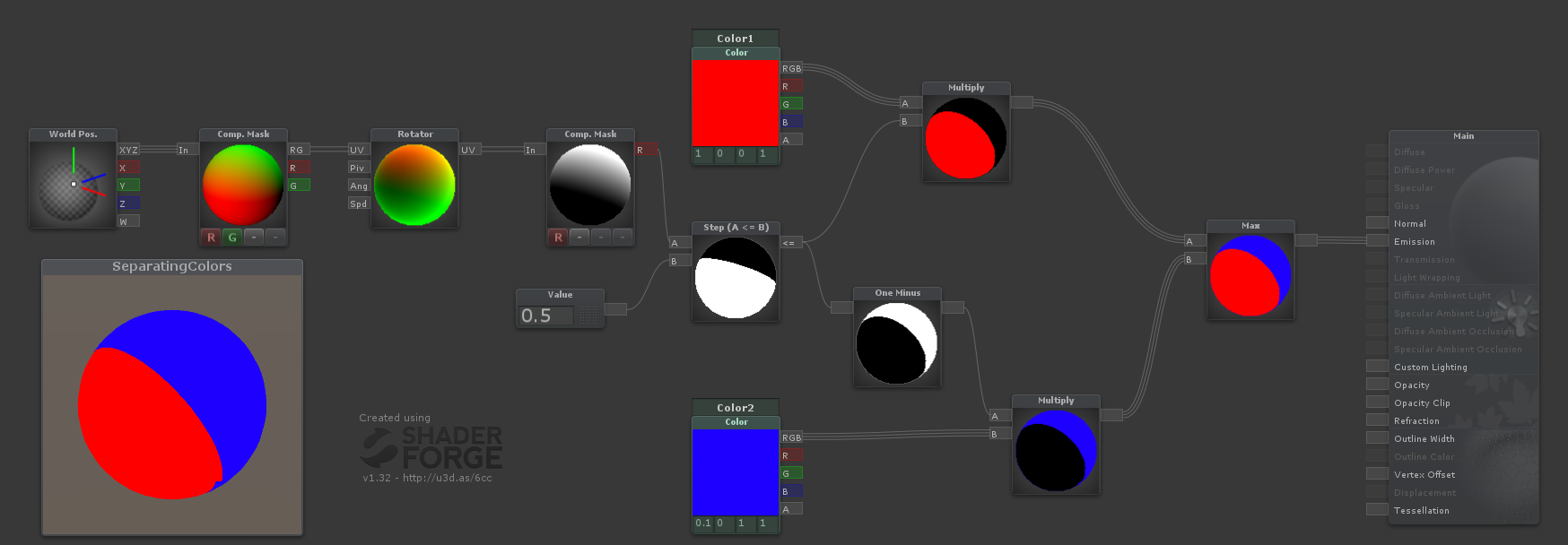# Color change effect, with a sharp line separating the old & new colors

I want to achieve some sort of "transformation" effect in Unity while changing colors in a mesh.

For instance, when changing its color from red to blue, I have a line going around the mesh, where the part of the mesh on one side of the line is red and the other is blue. Over time, the line moves so the object is entirely blue.

From what I understand, I would need some sort of custom shader to do that? Or would I need some sort of animation? I really have no clue how to achieve this kind of effect so any tips are welcome.

• You're saying you want the object to be red on one side of the line, blue on the other side of the line, and that line moves over time until the shape is entirely one colour? Do you have particular requirements on how the line wraps around the object's surface? (eg. the colour transition should start on this side / at this point, or should always be parallel to the horizontal plane, or...) – DMGregory Mar 10 '18 at 22:35
• Yes, that it but not real requirements on how it wraps around. I guess horizontal would be the best for what I want to achieve though. – Supaidaman Mar 11 '18 at 15:28
• Something like this image: imgur.com/a/mf0T8 – Supaidaman Mar 11 '18 at 15:34

This is pretty straightforward. I'll add it to a standard shader and comment on it as we go...Shader "Custom/TwoTone" {
Properties {
// Add a second color property
_Color ("Color", Color) = (1,1,1,1)
_Color2 ("Second Color", Color) = (1, 0, 0, 1)
_MainTex ("Albedo (RGB)", 2D) = "white" {}
_Glossiness ("Smoothness", Range(0,1)) = 0.5
_Metallic ("Metallic", Range(0,1)) = 0.0
// And a divider to store our transition plane
_Divider ("Divider", Vector) = (0, 1, 0, 1)
}
Tags { "RenderType"="Opaque" }
LOD 200

CGPROGRAM
#pragma target 3.0

sampler2D _MainTex;

struct Input {
float2 uv_MainTex;
float3 worldPos;
};

half _Glossiness;
half _Metallic;

UNITY_INSTANCING_BUFFER_START(Props)
// Color divider properties here for instancing, if desired.
fixed4 _Color;
fixed4 _Color2;
float4 _Divider;
UNITY_INSTANCING_BUFFER_END(Props)

void surf (Input IN, inout SurfaceOutputStandard o) {
// Compute the "height" of this point along the dividing axis.
float level = dot(IN.worldPos, _Divider.xyz);
// Select one of the two colours,
// depending on the threshold in the fourth component.
float thresh = sin(_Time.y) * 0.6f + 0.6f;
fixed4 color = lerp(_Color, _Color2, step(level, _Divider.w));

fixed4 c = tex2D (_MainTex, IN.uv_MainTex) * color;
o.Albedo = c.rgb;
o.Metallic = _Metallic;
o.Smoothness = _Glossiness;
o.Alpha = c.a;
}
ENDCG
}
FallBack "Diffuse"
}


The trick here is the _Divider property. The first three components of this vector, xyz represent the direction the line moves (the transition plane's normal vector). So by changing those you can make the split run top-to-bottom, left-to-right, whatever direction you need.

The last component w represents the height along that axis where the colour transition should happen. By sliding this value up and down, you move the transition plane.

Right now I'm doing the comparison in world space, but you can also do this in object space so the transition plane moves with the object rather than staying at a fixed spot in the world.

DMGregory answer Is correct but I like to explain It visually.

first we need a way to draw Black and White mask.since you need horizontal line we just use U in UVcoord,then we use step function to making thick line.It seems it's over but pay attention to below Image:as you can see uvcoordinate Isn't good choice here because It Isn't integrated and It Depends on the model's uvcoordinate.so Instead of It we can use world space .